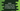# Kotlin program to check if an array contains any one of multiple values## Introduction :

In this tutorial, we will learn how to check if an array contains any one or another value in Kotlin. For example, if the array is 1, 2, 3, 4, 6, 8, if we check if it contains 1 or 3, then it should return true. Again, for the same array, if we check if it contains 10 or 11, it should return false because neither of these numbers exists in the array.

In Kotlin, we have different ways to do that. Let’s check them one by one :

### Using any :

any can be used to check one condition as a predicate in an array. It takes one predicate and returns one Boolean value based on the predicate. It is defined as below :

``fun <t> Array<t>.any(): Boolean``

We can call it directly on an array. For example :

``````fun main() {
val givenArray = intArrayOf(1, 2, 3, 4, 5, 7, 9, 11)

println(givenArray.any{ it == 5 || it == 12})
println(givenArray.any{ it == 5 || it == 11})
println(givenArray.any{ it == 15 || it == 21})
}``````

In this program, we have one predicate that checks if the current value is equal to one of two numbers. It will print the below output :

``````true
true
false``````

First two print statements will return true, but the third statement will return false because neither 15, nor 21 exists in the array.

### Using an array to store the values :

Instead of writing OR(||) for multiple values, we can also simplify it like below :

``````fun main() {
val givenArray = intArrayOf(1, 2, 3, 4, 5, 7, 9, 11)
val itemsArray = intArrayOf(5,11)

println(givenArray.any{ it in itemsArray})
}``````

It will check if any of the item in itemsArray is in givenArray or not. This method is more useful than the previous one because, we are keeping all in an array and checking if it exists from that array. With this method, we can add any number of items. Just add them in itemsArray and it will work.

### Using array contains :

We can also pass array.contains() method as a predicate. It will work like same :

``````fun main() {
val givenArray = intArrayOf(1, 2, 3, 4, 5, 7, 9, 11)
val itemsArray = intArrayOf(5,11)

println(givenArray.any(itemsArray::contains))
}``````

### Summary :

The below program puts everything in one place :

``````fun main() {
val givenArray = intArrayOf(1, 2, 3, 4, 5, 7, 9, 11)

println(givenArray.any{ it == 5 || it == 12})
println(givenArray.any{ it == 5 || it == 11})
println(givenArray.any{ it == 15 || it == 21})

val itemsArray = intArrayOf(5,11)
println(givenArray.any{ it in itemsArray})

println(givenArray.any(itemsArray::contains))
}``````

It will print :

``````true
true
false
true
true``````